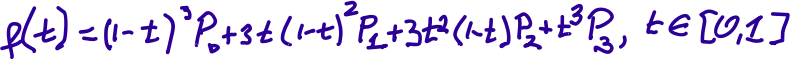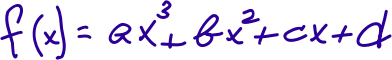## Cubic Bézier Equation

The function/expression/formula of the cubic Bézier curve used extensively in vector graphics, including SVG graphics used here. It expresses the same cubic curves as those written in the formula in the graphic this is a remix of, but it expresses them as a function of “time” (hence the use of f(t) rather than f(x) here). The time is not measured in seconds or any other units, as it is simply telling a browser or other software what order to turn on individual pixels to display the vector graphic on a raster device.

It moves from the point P to the point P, while using P and P as control points. It only does so for the values of t in the [0,1] set, i.e., for 0

Tags
Safe for Work?
Yes
•